Space Vector Quantities | Genesis Highspeed Data Recorder Space Vector Quantities | Genesis Highspeed Data Recorder | HBM

# Calculating Space Vector Quantities and Air-Gap Moment of Inverter-fed AC induction Motors Using Genesis HighSpeed and Perception Software

Genesis HighSpeed data recorders from HBM are particularly suitable for measurements on inverter-fed AC induction motors. Thanks to their modular design, these measurement systems allow any number of channels while providing high sampling rates. The electrical input quantities of electric motors can be precisely measured. UsingPerceptionsoftware, additional quantities such as magnetic flux or the motors' internal air-gap moment can be calculated.

## Introduction

Electrically driven vehicles are becoming increasingly important for individual mobility, since electric vehicles enable various renewable sources of energy to be easily used as propulsion energy . Fig. 1.1 shows the principle of an electric vehicle. The vehicle battery is charged from the mains through a charger. An inverter converts the battery's DC voltage to a three-phase alternating voltage and supplies an inverter-fed AC induction motor. Inverter-fed AC induction motors, synchronous, or reluctance motors can be used.

For technical and economical reasons, inverter-fed AC induction motors are often used in both industrial drives and vehicle drives. The energy stored in the battery determines the range of an electric vehicle. An energy capability of approximately 20 kWh/100 km is required, depending on the weight, driving style and quality of the route, as well as the efficiency of the drive components. The energy consumption and the required range of the vehicle determine the vehicle battery's properties such as capacitance, weight and cost. Efficient drive components are essential to increasing the range of electric vehicles.

In this paper, the functionality of the inverter-fed AC induction motor will be explained first. Then the mathematical description using space vectors will be introduced and implementation of the calculation method using Perceptiondescribed. The use of space vectors will be demonstrated through the example of real measurements using the GEN3i data recorder.

## Inverter-Fed AC Induction Motors

Due to their simple and robust design, inverter-fed AC induction motors are often used in traction drives. Fig. 2.1 shows a squirrel-cage type inverter-fed AC induction motors. The fixed stator has three windings. The squirrel-cage type is designed with bars made of aluminum or copper and is short-circuited with conductive rings at the rotor ends.

Applying three time-shifted currents to the spatially offset windings produces a rotating magnetic field. The magnetic field rotates with the synchronous rotational speed of: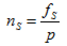(2.01)

Equation 2.01 shows that the synchronous rotational speed depends on the stator currents' frequency fS and the inverter-fed AC induction motor's so-called number of pole pairs p.

The magnetic field runs through the squirrel-cage rotor. This motion induces voltages in the conductive bars. Due to the shorting rings at the rotor ends, large currents resulting from the induced voltage flow in the bars. According to Lenz's law, forces are produced that accelerate the rotor in the direction of the rotating field. This reduces the difference in rotational speed between the rotor and the rotating field.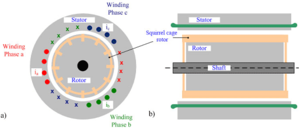Fig. 2.1: Structure of an inverter-fed AC induction motor with squirrel-cage rotor

a) Cross section and b) longitudinal section of the motor

### Space Vectors

In 1959, Kovacs developed the space vector theory to facilitate the mathematical description of three-phase systems. It is often used in practice to describe control methods for inverter-fed AC induction motors.  The electric and magnetic parameters of a three-phase system can be mapped on a two-phase, orthogonal system and a zero-sequence system that is present in certain circumstances. The two-phase, orthogonal system can be interpreted as a complex number called space vector. Its real and imaginary parts correspond to the projections of the complex number, displayed as a vector, on the α and β axes in the complex plane. Equation 2.02 defines the calculation rules for the complex space Vectorfrom the three phase quantities x1, x2 and x3 .(2.02)

with α being a complex operator. The associated zero-sequence system is calculated as follows: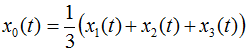(2.03)

Figure 2.2 shows space vectorin the orthogonal coordinate system. The space vector's real part is plotted on the abscissa α and the imaginary part on the ordinate β. The phase quantities are obtained by projecting the space vector on the axes a,b,c rotated by 120°.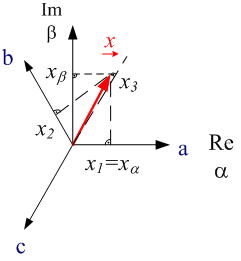Fig. 2.2: Space vectors in the complex plane

### Equivalent circuit diagram of an inverter-fed AC induction motor using space vectors

The operating behavior of electric motors is often described by equivalent circuit diagrams. Fig. 2.3 shows the simplified equivalent circuit diagram of an inverter-fed AC induction motor using space vectors. The stator voltage applied to the  inverter-fed AC induction motor via the inverter is described by space vector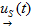. Stator current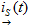flows into the inverter-fed AC induction motor. In the equivalent circuit diagram, the stator resistance is represented by Rs. Inductance Lμ is the motor's magnetization inductance. The motor's leakage inductances are combined in Lσ. Ry designates the rotor resistance converted to the stator side. The mechanical rotational speed n at the inverter-fed AC induction motor's shaft is accounted for in the equivalent circuit diagram through the electromechanical angular frequency ω. These two quantities can be converted into each other through the number of pole pairs ρ.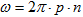(2.04)

For more detailed information about the inverter-fed AC induction motor, please refer to .Fig. 2.3: Simplified equivalent circuit diagram of an inverter-fed AC induction motor.

## Measurements on an Inverter-Fed AC Induction Motor

Fig. 3.1 shows the time behavior of an inverter-fed AC induction motor's phase voltages (u1(t),u2(t),u3(t)) and phase currents (i1(t),i2(t),i3(t)). The currents and voltages' counting direction is shown in the overview in figure 1.1. The space vector quantities can be calculated from the measured phase quantities using equation 2.02. Due to the fact that in Perception all variables need to be real numbers, the space vector's real and imaginary parts are calculated separately. The following equation shows this calculation for the phase voltage and the phase current.(3.01)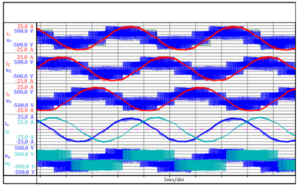Fig. 3.1: Measured phase quantities (i1,i2,i3,u1,u2,u3) and calculated space vector quantities (ia,ib,ua,ub) (For Perception users, these measurements are available in the following file: Space Vector and Air GapTorque .pNRF. This file is available for download from the HBM website).

### Calculating the stator flux of an inverter-fed AC induction motor

The stator flux space vector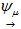describes the magnetic field in an inverter-fed AC induction motor. The stator flux space vector is obtained by integrating the difference between stator voltage and voltage drop at the motor's stator resistance Rs.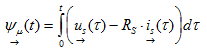(3.06)

The often low stator resistance can be neglected with some motors, depending on the accuracy requirements. As a result of the integration, the stator flux is a continuous quantity. Even though, as shown in Fig. 3.1, the stator voltage shows pulse width modulated behavior, the stator flux space vector's real ψα(t) and imaginary parts ψβ(t) are approximately sinusoidal (Fig. 3.2a). As a rough approximation, the stator flux space vector's trajectory follows a circular path. The radius of the circular path corresponds to the amplitude of the stator flux.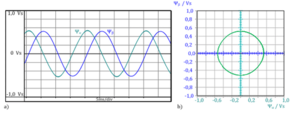Fig. 3.2:
Stator flux space vector of the inverter-fed AC induction motor
a) Time behavior of the real and imaginary parts
b) Trajectory of the space vector in the complex plane

### Calculating the Internal Air-Gap Moment of an Inverter-Fed AC Induction Motor

With inverter-fed AC induction motors, the so-called internal or air-gap moment can be calculated from voltage or flux and current measurements. The internal air-gap moment consists of the motor's internal inevitable friction torques and the torque at the shaft. Ignoring frictional torque, the calculated internal torque corresponds to the mechanical torque which can be measured very precisely using a torque transducer .

The accuracy of the calculated torque depends on the calculation model used and the accuracy of its motor parameters. The calculation of the internal torque can be used as redundant information complementing a torque transducer's measurement signal, because its amount should at least be on the same order of magnitude as the mechanical moment. Moreover, the proportional torque of an electric motor can be correlated with the entire drive train's torque measured using the torque transducer. A potential application is, for example, a hybrid vehicle, because its combustion engine and electric motor act on the same drive train. With the calculated torque of the electric motor, the moment of the combustion engine can be determined.

It is known from the literature  that the internal torque of inverter-fed AC inductionmotors can be calculated from the stator currents and magnetic stator fluxes.(3.06)

Here, ρ represents the inverter-fed AC induction motor's number of pole pairs. Currents and fluxes are represented by their space vector components. Fig. 3.3 shows the time behavior of the stator currents and the fluxes, as well as the internal air-gap moment Mi calculated from these values. The resulting torque ripple is clearly visible. High-frequency torque ripple is generated as a result of the inverter's switching operation. It is essential to correctly identify the period of the motor current's fundamental to enable the air-gap moment to be calculated in Perception.Fig. 3.3: Time behavior of stator current (iα, iβ), stator flux (ψα, ψβ) and the calculated air-gap moment Mi

## Conclusions

This paper presents measurements on an inverter-fed AC induction motor. The measured phase quantities are converted into so-called space-vector quantities to facilitate analysis of the measurement results. The magnetic flux in the inverter-fed AC induction motor is calculated through integration of the stator voltage. These electrically measured quantities combined with the calculated magnetic quantities enable the inverter-fed AC induction motor's internal air-gap moment to be calculated. This torque calculation provides a redundant measurement signal complementing the signal from a high-precision torque transducer. The calculated torque value allows one to carry out a plausibility check of the measurement and to quickly identify measurement errors.

### References

 D. Eberlein; K. Lang; J. Teigelkötter; K. Kowalski: Elektromobilität auf der Überholspur: Effizienzsteigerung für den Antrieb der Zukunft; Tagungsband 3. Tagung Innovation Messtechnik; 14. Mai 2013

 J. Teigelkötter: Energieeffiziente elektrische Antriebe, 1. Auflage, Springer Vieweg Verlag, 2013; ISBN 3-8348-1938-3

 R. Schicker; G. Wegener: Drehmoment richtig messen; Hottinger Baldwin Messtechnik GmbH 2002, ISBN 3-00-008945-4

 Berechnung von Leistungsgrößen mit Perception-Software                                                                                                                     https://www.hbm.com/de/3783/berechnung-von-leistungsgroessen-mit-perception-software/
 www.hbm.com